# 7.3 SOME PROPERTIES Of A TRIANGLE:

*  Sides opposite to equal angles are equal.

*  Angles opposite to equal sides are equal.

*  A triangle in which two sides are equal is called an isosceles triangle.

*  Two figures are congruent it they are of the same shape and size.

*  In a triangle the angle opposite to the larger side is greater.

*  Sum of two sides of a triangle is always greater than the third side.

*  Two angles are congruent only when their measures are equal.

Exercise 7.2

1. In an isosceles triangle ABC, with AB = AC, the bisectors of ∠B and ∠C intersect each other at O. Join A to O. Show that:   (i)  OB = OC       (ii)  AO bisects ∠A.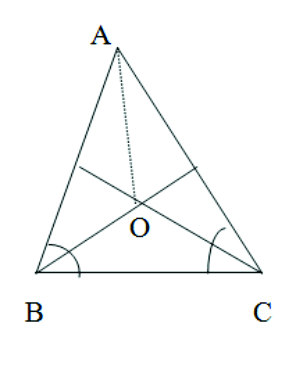Given: AB = AC   and  the bisectors of ∠B and ∠A meet at O
To prove:    (i) OB = OC      (ii)  AO bisects ∠A
Proof:  (i) AB = AC     (given)
∴  ∴ ∠C = ∠B        (angles opposite to equal sides are equal )
∴    1/2 ∠C   =   1/2 ∠B
∴   ∠OCB   =  ∠OBC
∴   OB  =  OC      (sides opposite to equal angles are equal)

(ii) In Δ ABO   and   Δ ACO   we have
AB = AC     (given)
OB = OC     (proved)

∠OBA = ∠OCA       (1/2∠B  = 1/2∠C)

By S.A.S congruence rule    Δ ABO   ≅  Δ ACO
∴   ∠OAB = ∠OAC      (c.p.c.t)

∴   AO   bisects ∠A

2. In Δ ABC, AD is the perpendicular bisector of BC . Show that Δ ABC is an isosceles triangle in which AB = AC.Given: AD is perpendicular bisector means   BD = CD and

To prove:   AB = AC

Proof: In Δ ABD   and  Δ ACD we have

BD = CD          (given)

By S.A.S congruence rule

⊿ABD ≅⊿ACD

∴ Their corresponding parts are equal

∴  AB  =  AC      (c.p.c.t)

∴    ΔABC is an isosceles triangle.

3.  ABC is an isosceles triangle in which altitudes BE and CF are drawn to equal sides AC and AB respectively . Show that these altitudes are equal.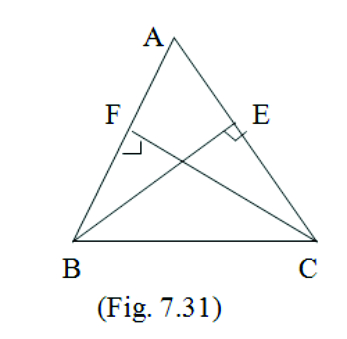Given: ABC is an isosceles triangle   i.e:    AB = AC

To prove: BE = CF

Proof:  Since AB = AC               (given)

∠ACB = ∠ABC     (Angles opposite to equal sides are equal)

Now in    Δ BEC   and   Δ CFB   we have

∠EBC = ∠FBC     (proved above)

BC = BC         (common side)
∠BEC   = ∠CFB        ( each  = 900 )

By S.A.S congruence rule

Δ BEC   ≅  Δ CFB

Their corresponding parts are equal

BE = CF          (c.p.c.t)

4. ABC is a triangle in which altitudes BE and CF to AC and AB are equal. Show that (i)  Δ ABE  ≅ Δ ACF           (ii)  AB   =  AC,    i.e. ABC is an isosceles triangle.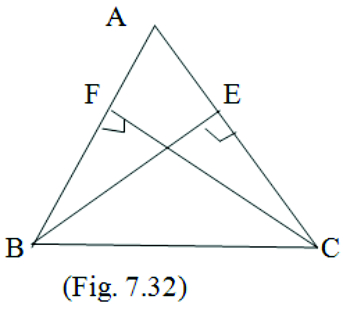Given:   BE  = CF

To prove:  (i)  ΔABE   ≅  ΔACF    (ii) AB = AC

Proof:  In Δ ABE and Δ ACF   we have

Δ AEB  =  Δ AFC   (each  = 900 )

A = A         (common angle)

BE = CF     (given)

By A.A.S congruence rule

Δ ABE   ≅  Δ ACF

(ii)  Their corresponding parts are equal

AB = AC (c.p.c.t)

ABC is an isosceles triangle

5. ABC and DBC are two isosceles triangles on the same base BC .
Show that ΔABD  ≅  ΔACD.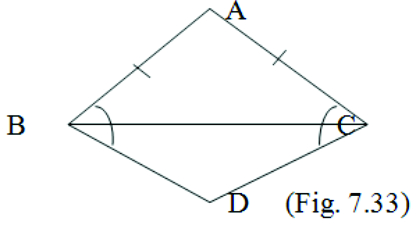Solution:

Given:  AB = AC   and   BD = CD

To prove: Δ ABD  ≅  Δ ACD

Proof:  In ΔABC we have

AB = AC    ( ΔABE is isosceles)

∠ABC = ∠ACB

(angles opposite to equal sides are equal)  —– (1)

Now in ΔBDC we have

BD = CD                    ( Δ BDC is isosceles)

∠CBD   =  ∠BCD       (angles opposite to equal sides are equal)  —– (2)

Adding (1) and (2) we get

Δ ABC   ≅  Δ ACB

6. ABC is an isosceles triangle in which AB = AC. Side BA is produced to D such that AD = AB .
Show that  ΔBCD is a right triangle.

Given : AB = AC and AD = AB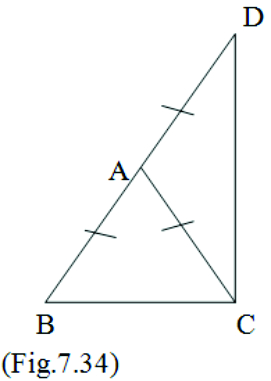To prove : ∠BCD = 900

Proof:  In   Δ ABC we have

AB = AC        (given)    ——- (1)

AD = AB        (given)    ——- (2)

From (1) and (2) we get

Now in   Δ ABC we have
∠ABC + ∠ACB + ∠BAC  = 1800       (angles of a Δ = 1800)
∠ACB + ∠ACB + ∠BAC  = 1800
(∠ABC = ∠ACB because they are angles opposite to equal sides)

∴  2 ∠ACB  + ∠ BAC = 1800   —— (3)

Now in  Δ  ACD   we  have

∠ADC + ∠ACD + ∠CAD  = 1800     (angles of a Δ= 1800)

∠ACD + ∠ACD + ∠CAD =  1800            ( ∠ADC = ∠ACD because they are angles opposite to equal sides)

∴  2 ∠ACD + ∠CAD  =  1800   —— (4)

Adding (3) and (4) we get

2 ∠ACB + ∠BAC + 2 ∠ACD + ∠CAD  =  1800 + 1800

2 ( ∠ACB + ∠ACD) + ∠BAC + ∠CAD  =  3600

2 (∠ACB + ∠ACD) +   1800    =  3600    ( ∠BAC + ∠CAD = 1800 because it forms a linear pair)

2 (∠ ACB + ∠ACD)                = 3600  – 1800

2 ( ∠ACB + ∠ACD)              = 1800

2 ∠BCD           = 1800              ( ∠ACB + ∠ACD  = ∠BCD)

∠BCD           = 1800  / 2

∠BCD            = 900

7. ABC is a right angled triangle in which A = 900   and   AB  =  AC.    Find  ∠B  and  ∠C.

Given
: ∠A = 90°  and  AB = AC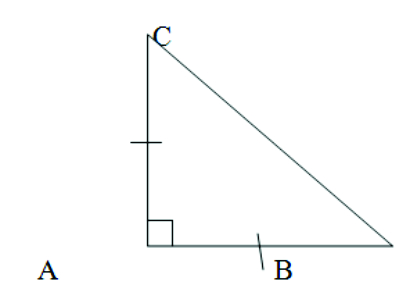To prove : ∠B = ?   ∠C =  ?

Proof:  AB = AC (given)

∠ACB = ∠ABC   (angles opposite to equal sides are equal)

We know that in Δ ABC

∠A + ∠B + ∠C = 1800     ( angles of a  Δ  = 1800)

900 +∠ B + ∠C = 1800

∠B + ∠C = 1800 – 900

∠B + ∠C =  900

Since AB = AC     we get    ∠B = ∠C

∴ ∠B + ∠B =  900

2 ∠B =  900

∠B =  450

And  ∠C + 450 =  900

∠C =  450

∠B =  450 and ∠C = 450

8. Show that the angles of an equilateral triangle are 600 each.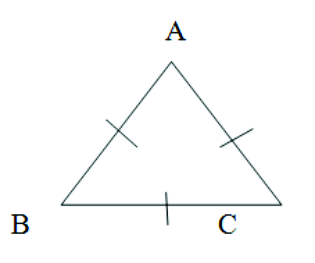Given: AB = CA = BC

To prove : ∠A = ∠B = ∠C = 600

Proof:  Since AB = BC  we get

∠C = ∠A     (angles opposite to equal sides are equal) ——(1)

And since AC = BC   we get

∠B = ∠A   (angles opposite to equal sides are equal) ——(2)

From (1) and (2) we get

∠A = ∠B = ∠C

Let  ∠A = ∠B = ∠C = ∠x

∠A + ∠B + ∠C = 1800       (angles of a  Δ = 1800)

∠x  +  ∠x  +  ∠x  = 1800

3∠x  =  1800

∠x = 1803 = 600

∠A = ∠B = ∠C = 600

Thus the angles of an equilateral triangle are 600 each.